Скачать презентацию Agenda Day 1 8 00 am

ca919dee9a5b642aa7d1b50a8121fbd8.ppt

• Количество слайдов: 39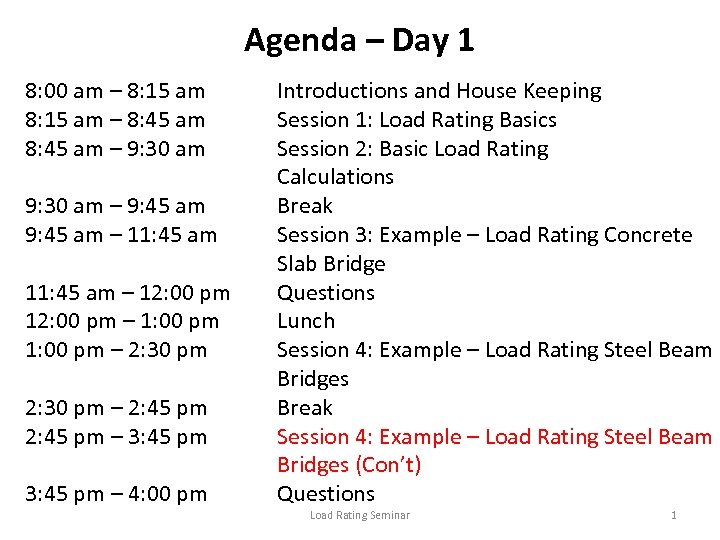Agenda – Day 1 8: 00 am – 8: 15 am – 8: 45 am – 9: 30 am – 9: 45 am – 11: 45 am – 12: 00 pm – 1: 00 pm – 2: 30 pm – 2: 45 pm – 3: 45 pm – 4: 00 pm Introductions and House Keeping Session 1: Load Rating Basics Session 2: Basic Load Rating Calculations Break Session 3: Example – Load Rating Concrete Slab Bridge Questions Lunch Session 4: Example – Load Rating Steel Beam Bridges Break Session 4: Example – Load Rating Steel Beam Bridges (Con’t) Questions Load Rating Seminar 1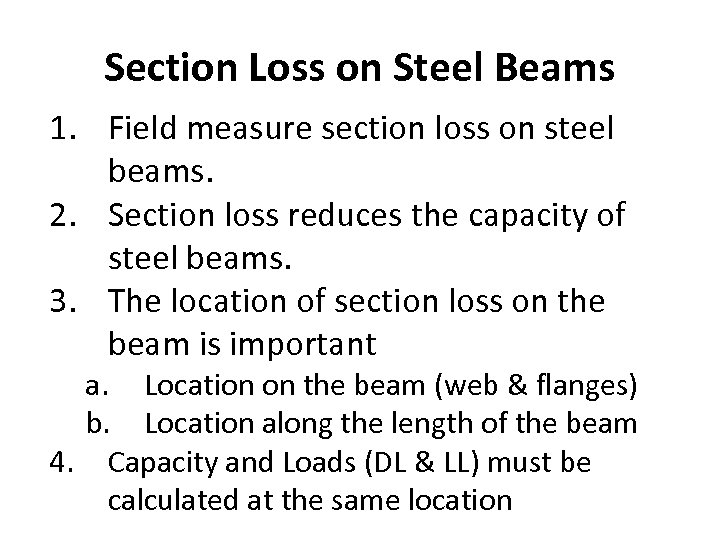Section Loss on Steel Beams 1. Field measure section loss on steel beams. 2. Section loss reduces the capacity of steel beams. 3. The location of section loss on the beam is important a. Location on the beam (web & flanges) b. Location along the length of the beam 4. Capacity and Loads (DL & LL) must be calculated at the same location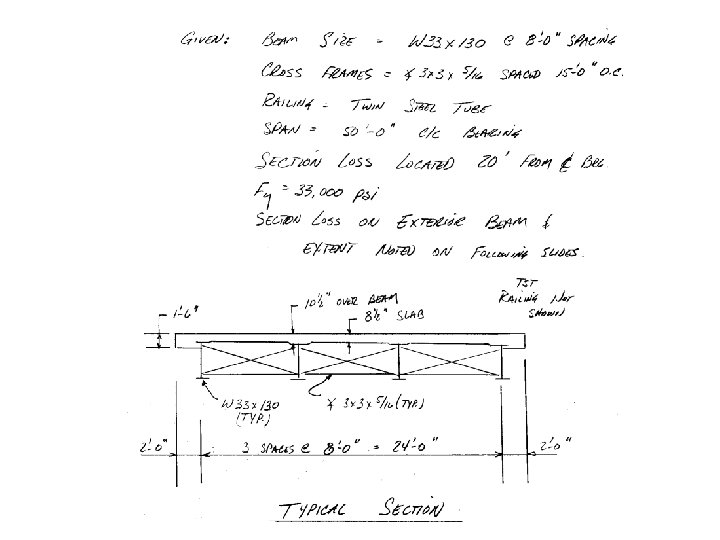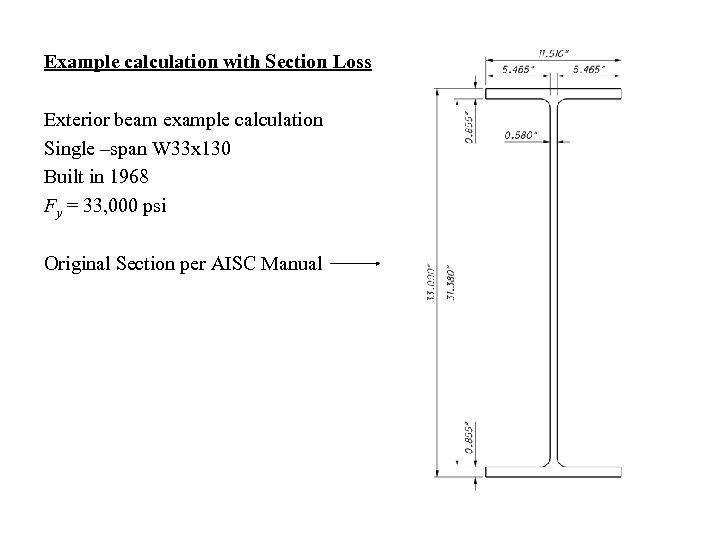Example calculation with Section Loss Exterior beam example calculation Single –span W 33 x 130 Built in 1968 Fy = 33, 000 psi Original Section per AISC Manual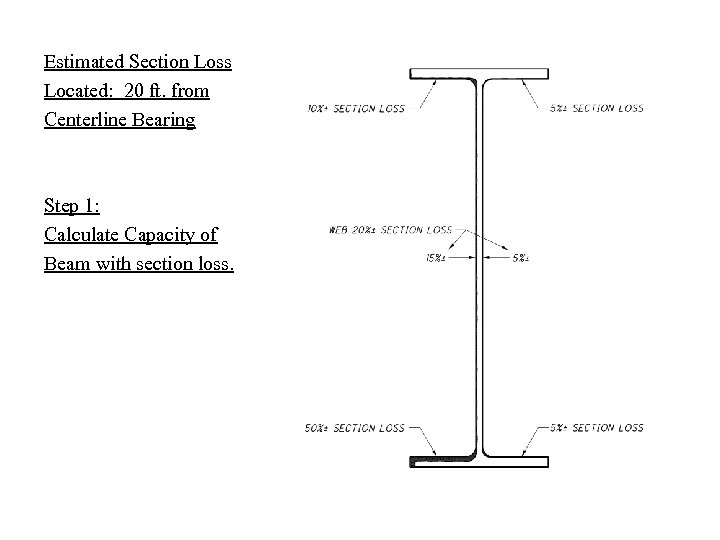Estimated Section Loss Located: 20 ft. from Centerline Bearing Step 1: Calculate Capacity of Beam with section loss.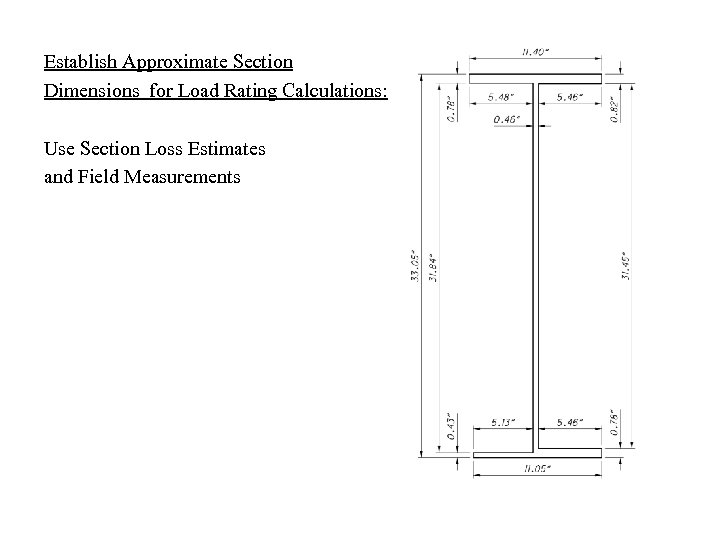Establish Approximate Section Dimensions for Load Rating Calculations: Use Section Loss Estimates and Field Measurements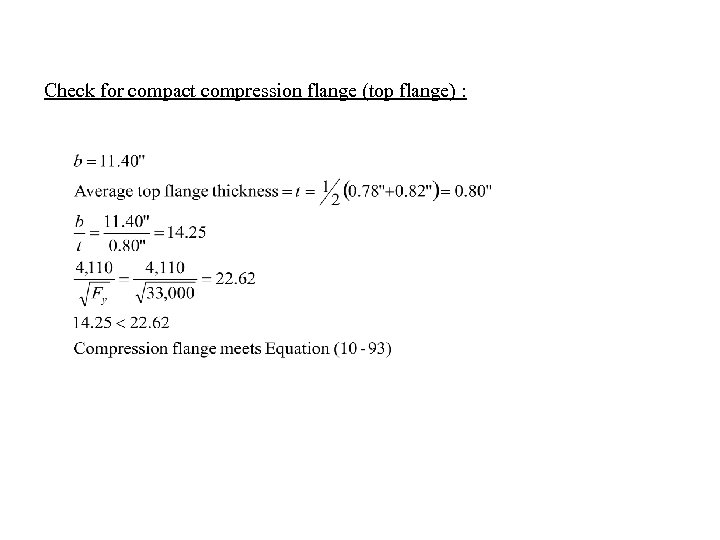Check for compact compression flange (top flange) :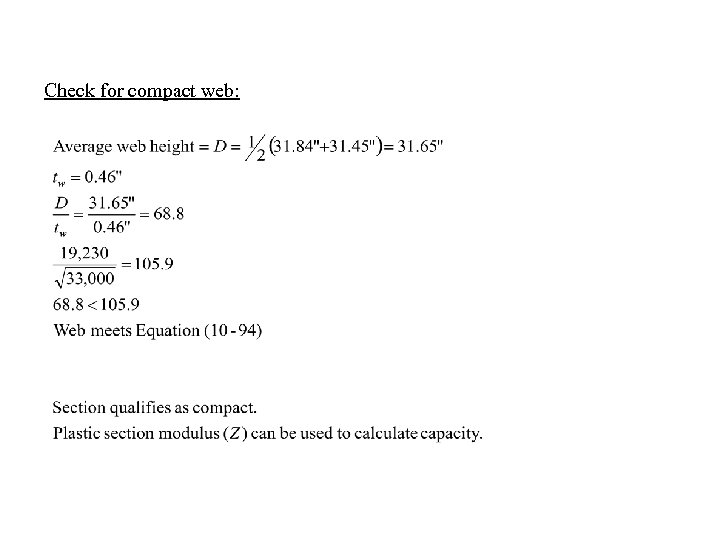Check for compact web: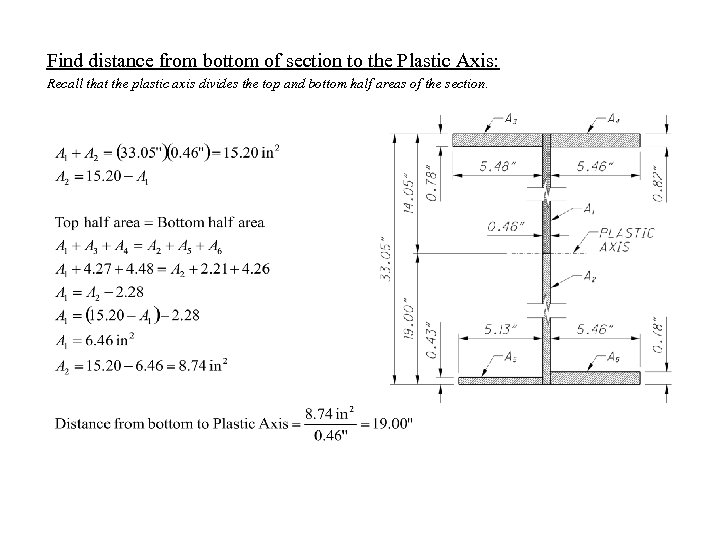Find distance from bottom of section to the Plastic Axis: Recall that the plastic axis divides the top and bottom half areas of the section.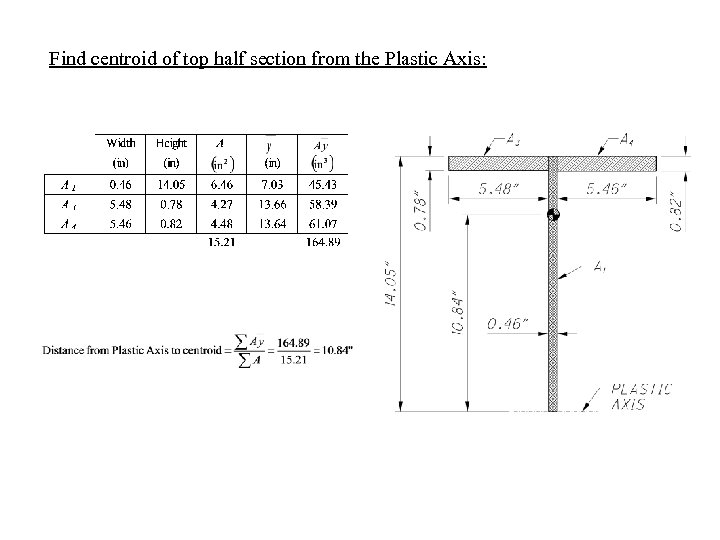Find centroid of top half section from the Plastic Axis: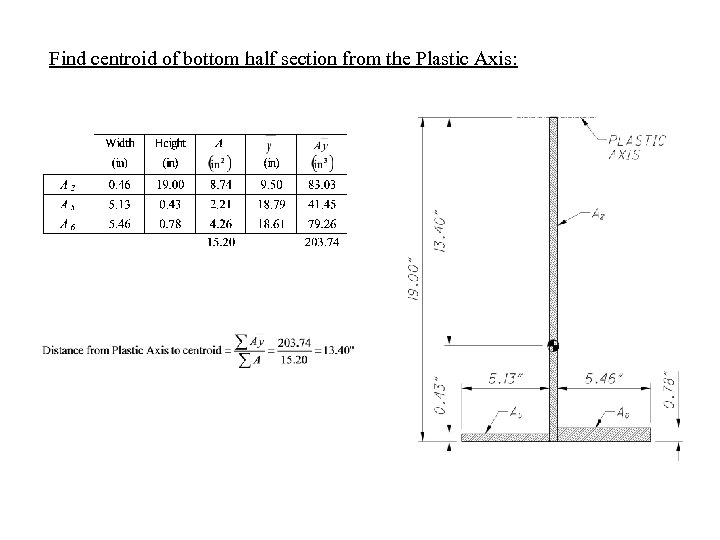Find centroid of bottom half section from the Plastic Axis: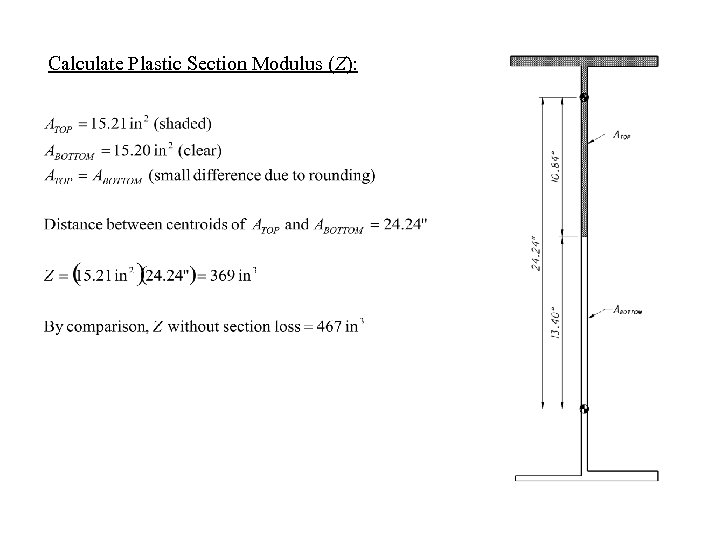Calculate Plastic Section Modulus (Z):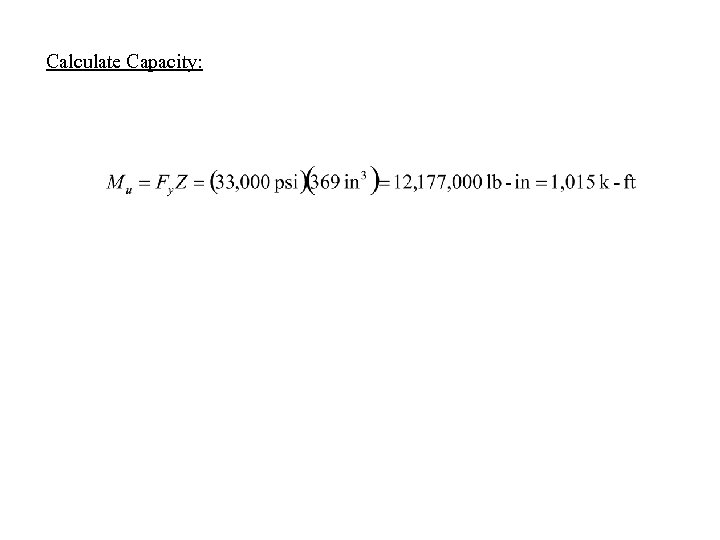Calculate Capacity: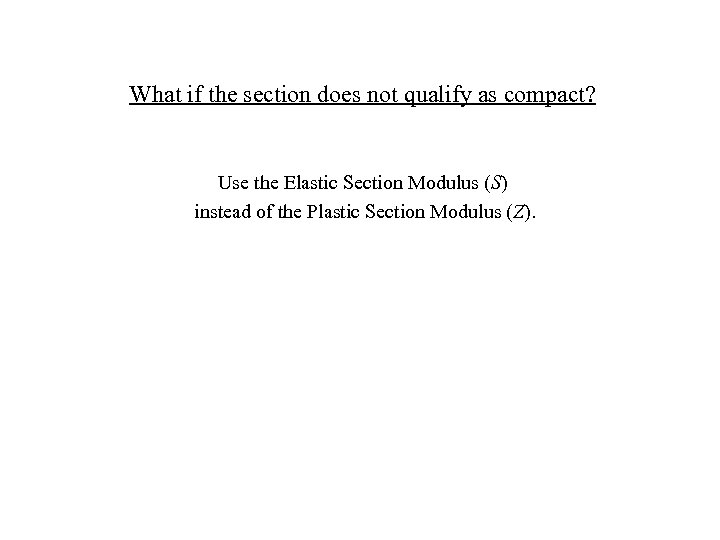What if the section does not qualify as compact? Use the Elastic Section Modulus (S) instead of the Plastic Section Modulus (Z).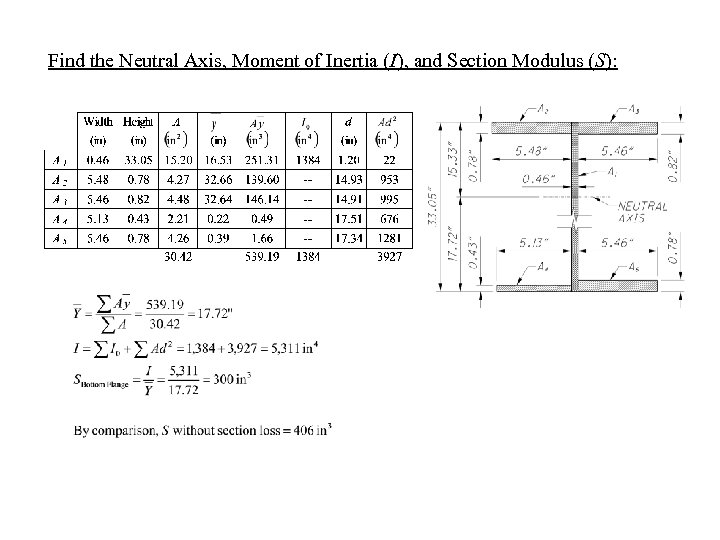Find the Neutral Axis, Moment of Inertia (I), and Section Modulus (S):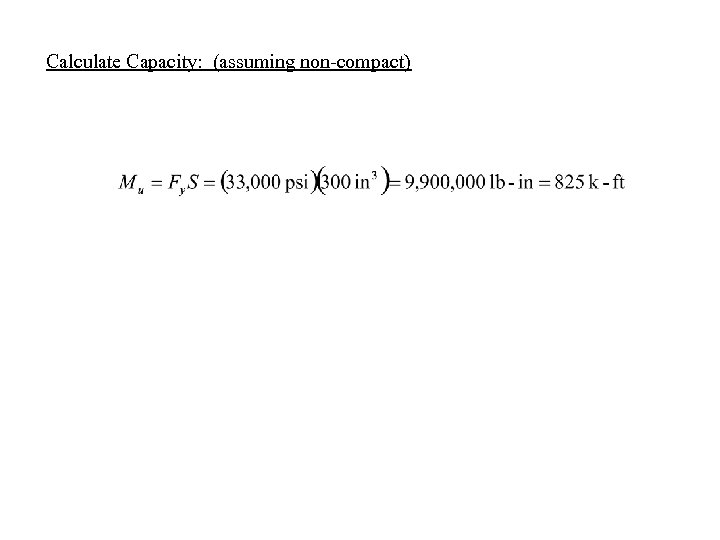Calculate Capacity: (assuming non-compact)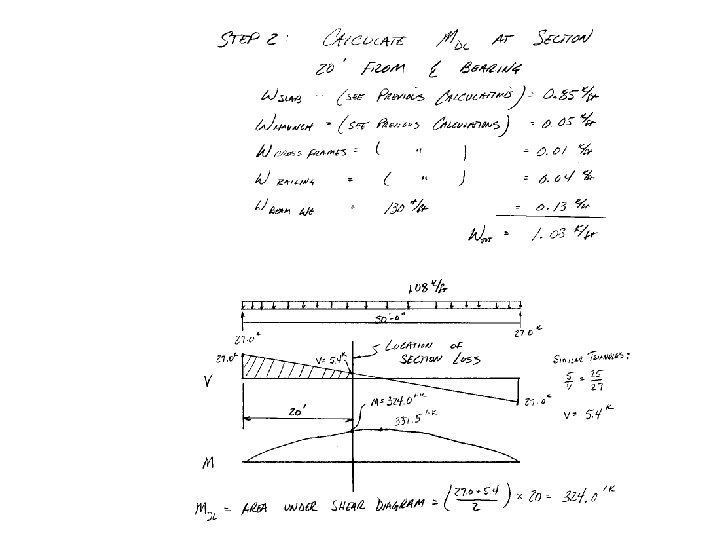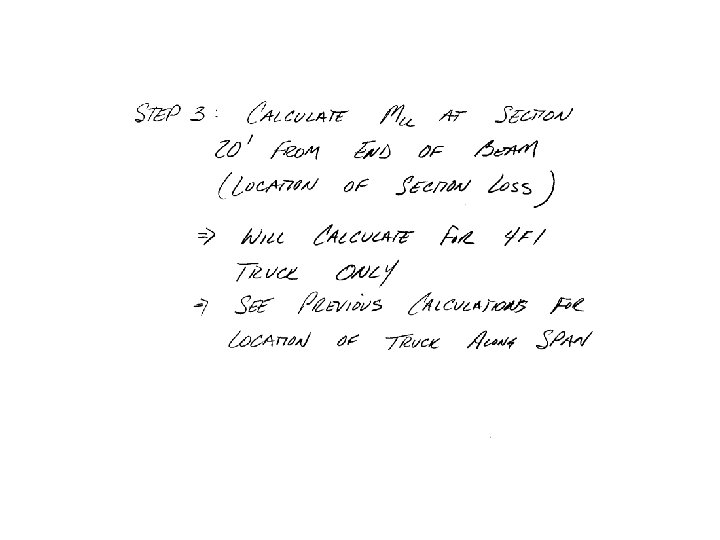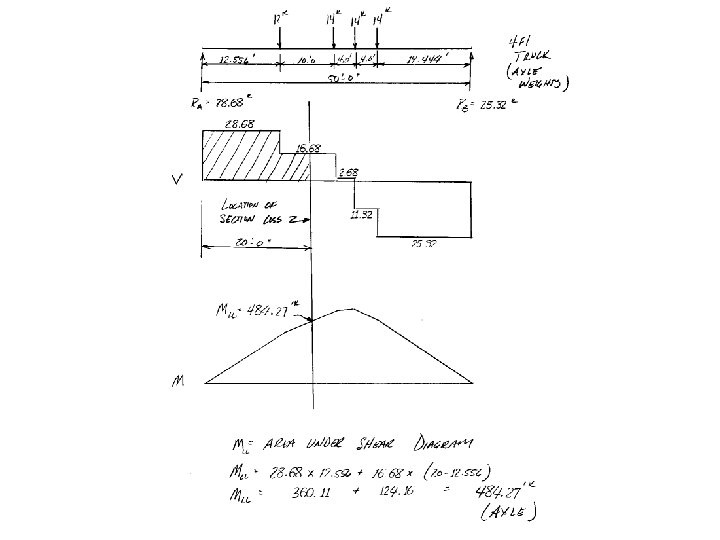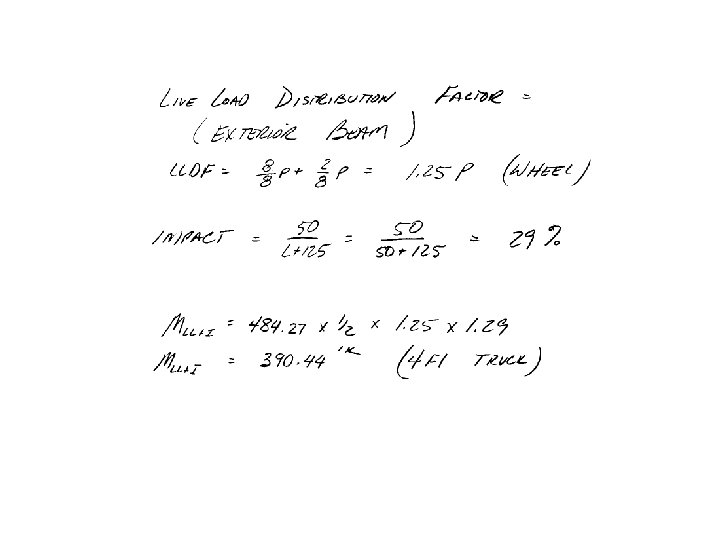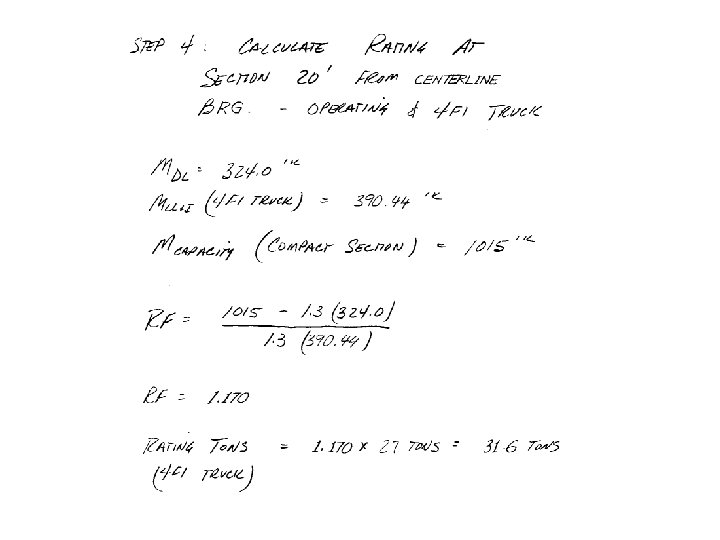Questions ? ?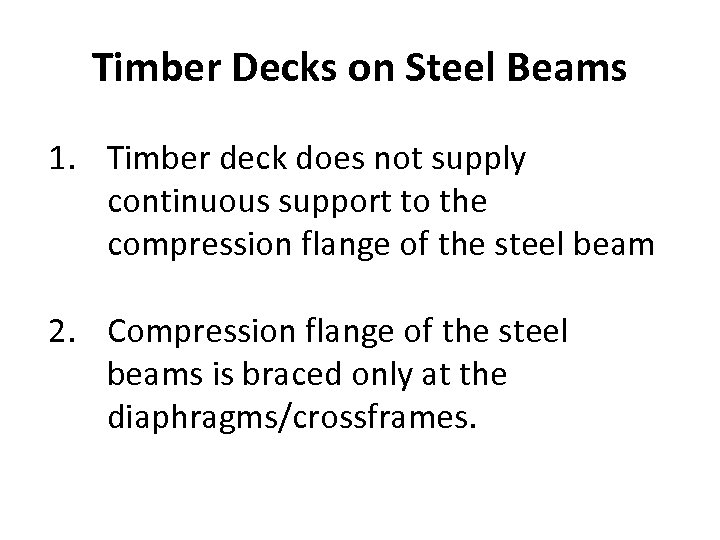Timber Decks on Steel Beams 1. Timber deck does not supply continuous support to the compression flange of the steel beam 2. Compression flange of the steel beams is braced only at the diaphragms/crossframes.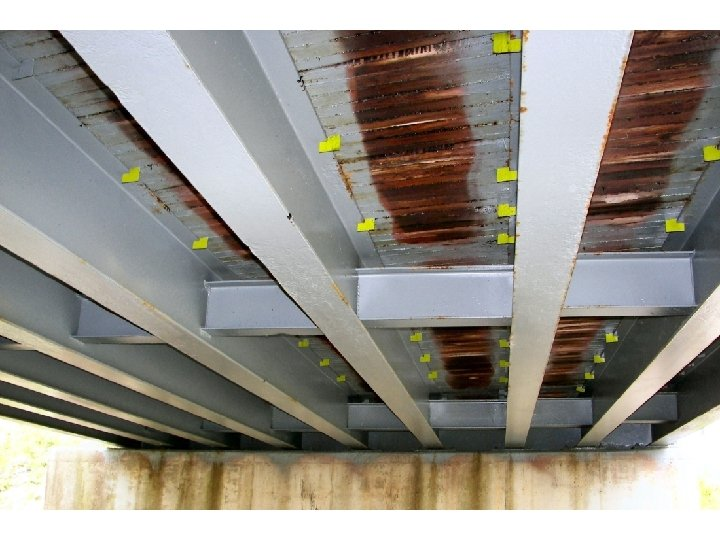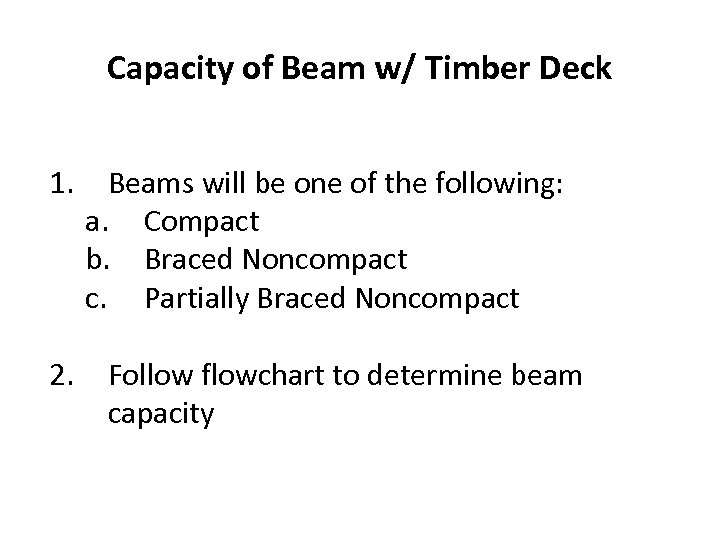Capacity of Beam w/ Timber Deck 1. 2. Beams will be one of the following: a. Compact b. Braced Noncompact c. Partially Braced Noncompact Follow flowchart to determine beam capacity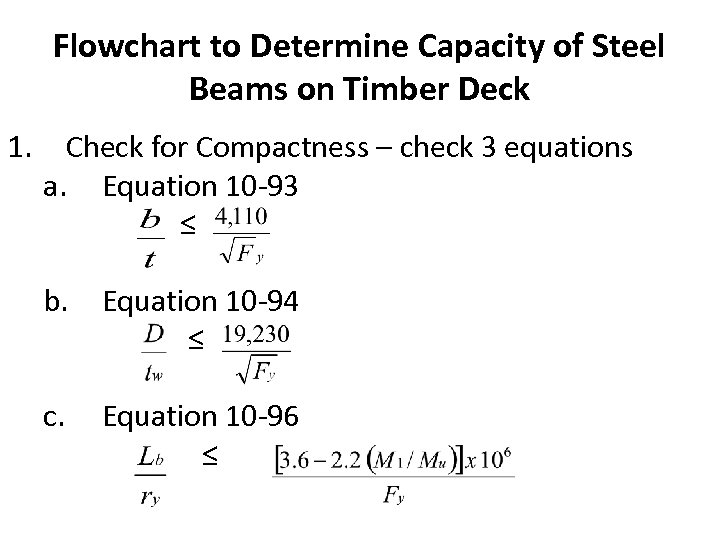Flowchart to Determine Capacity of Steel Beams on Timber Deck 1. Check for Compactness – check 3 equations a. Equation 10 -93 ≤ b. Equation 10 -94 ≤ c. Equation 10 -96 ≤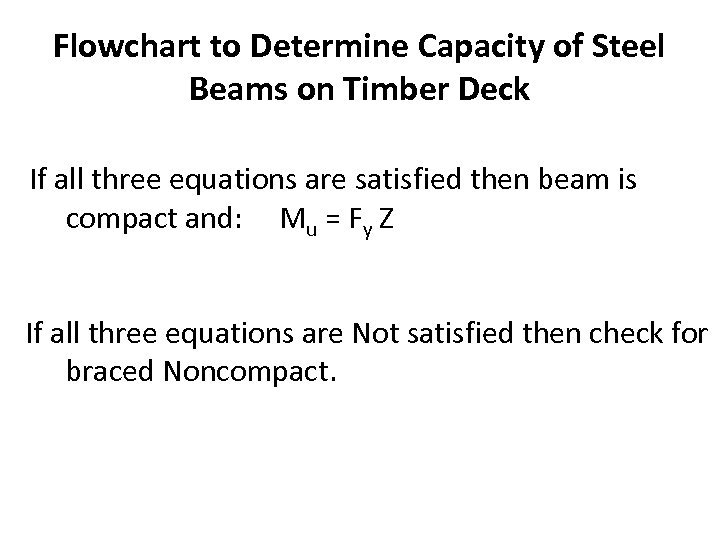Flowchart to Determine Capacity of Steel Beams on Timber Deck If all three equations are satisfied then beam is compact and: Mu = Fy Z If all three equations are Not satisfied then check for braced Noncompact.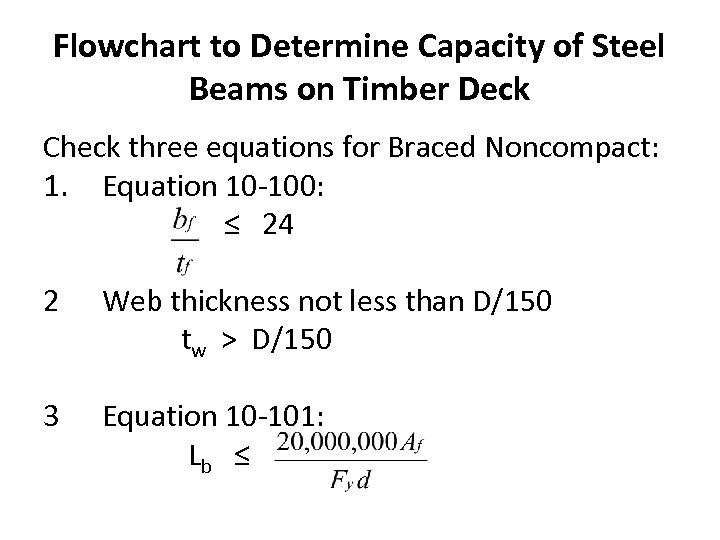Flowchart to Determine Capacity of Steel Beams on Timber Deck Check three equations for Braced Noncompact: 1. Equation 10 -100: ≤ 24 2 Web thickness not less than D/150 tw > D/150 3 Equation 10 -101: Lb ≤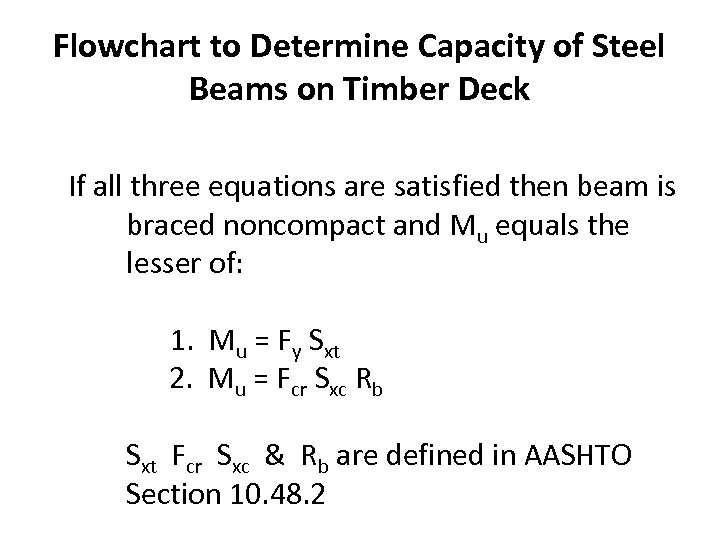Flowchart to Determine Capacity of Steel Beams on Timber Deck If all three equations are satisfied then beam is braced noncompact and Mu equals the lesser of: 1. Mu = Fy Sxt 2. Mu = Fcr Sxc Rb Sxt Fcr Sxc & Rb are defined in AASHTO Section 10. 48. 2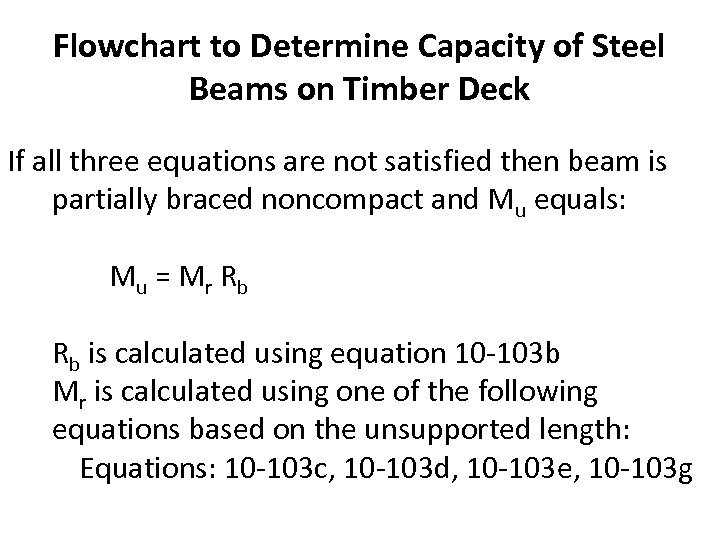Flowchart to Determine Capacity of Steel Beams on Timber Deck If all three equations are not satisfied then beam is partially braced noncompact and Mu equals: M u = Mr Rb Rb is calculated using equation 10 -103 b Mr is calculated using one of the following equations based on the unsupported length: Equations: 10 -103 c, 10 -103 d, 10 -103 e, 10 -103 g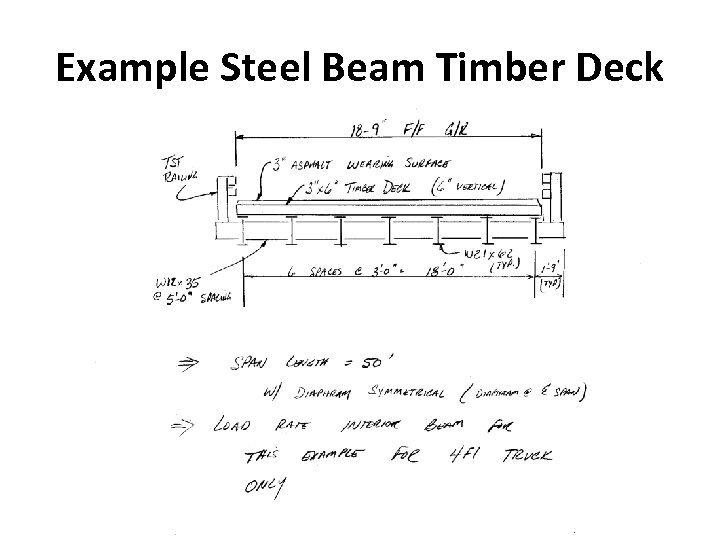Example Steel Beam Timber Deck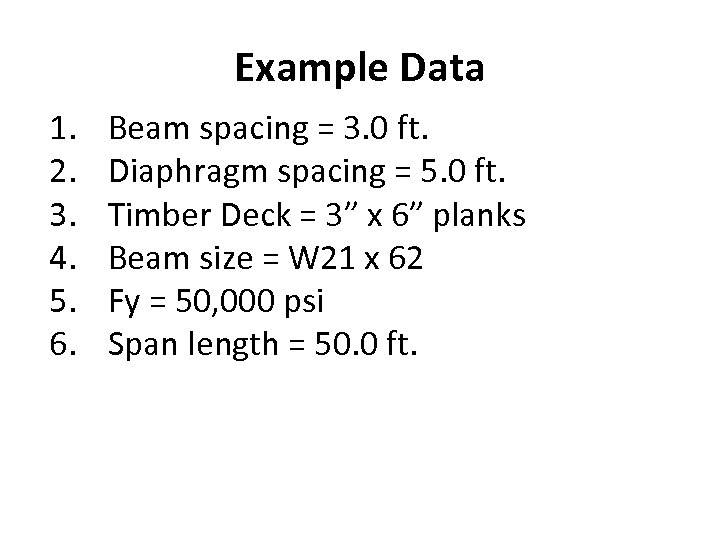Example Data 1. 2. 3. 4. 5. 6. Beam spacing = 3. 0 ft. Diaphragm spacing = 5. 0 ft. Timber Deck = 3” x 6” planks Beam size = W 21 x 62 Fy = 50, 000 psi Span length = 50. 0 ft.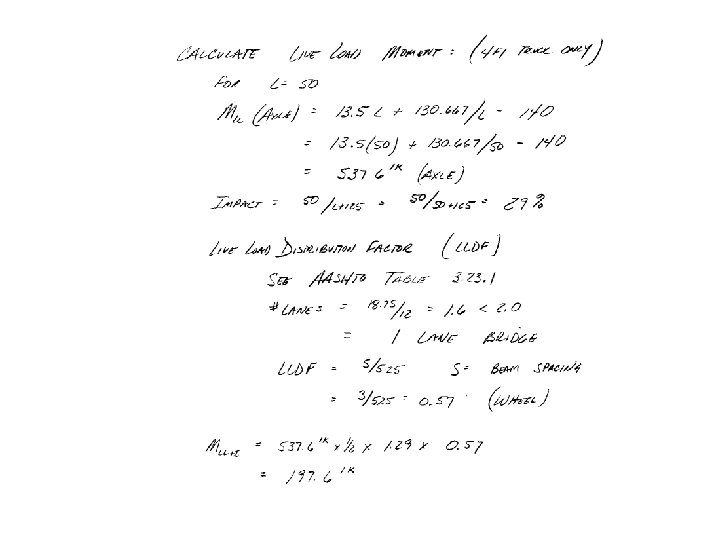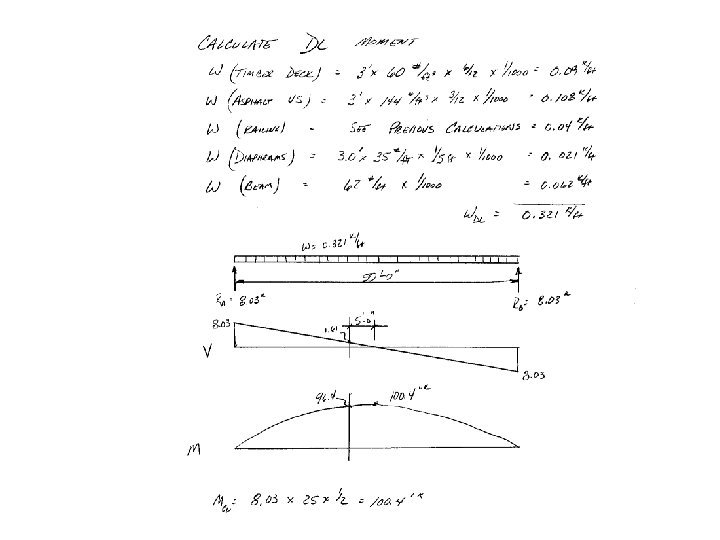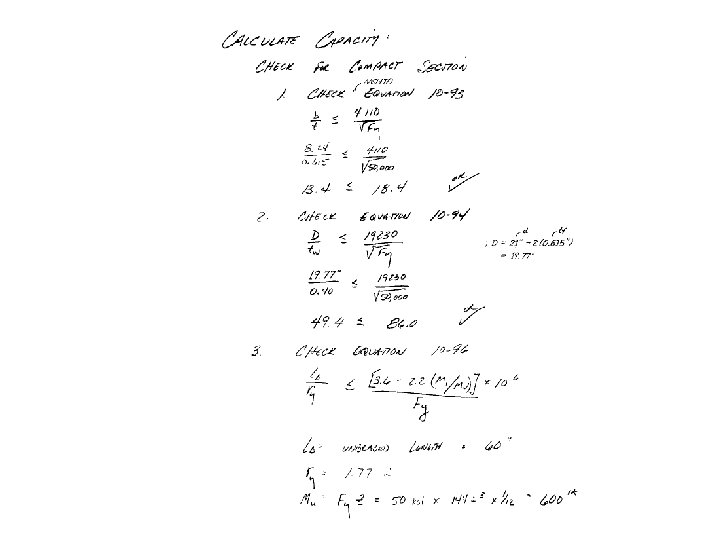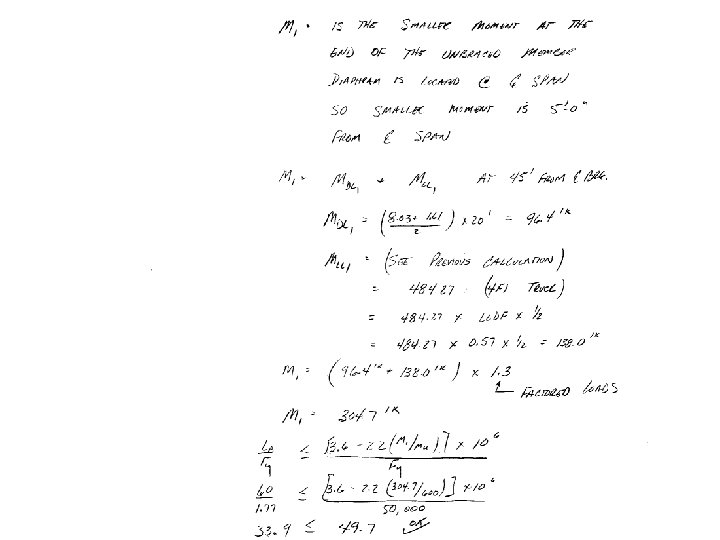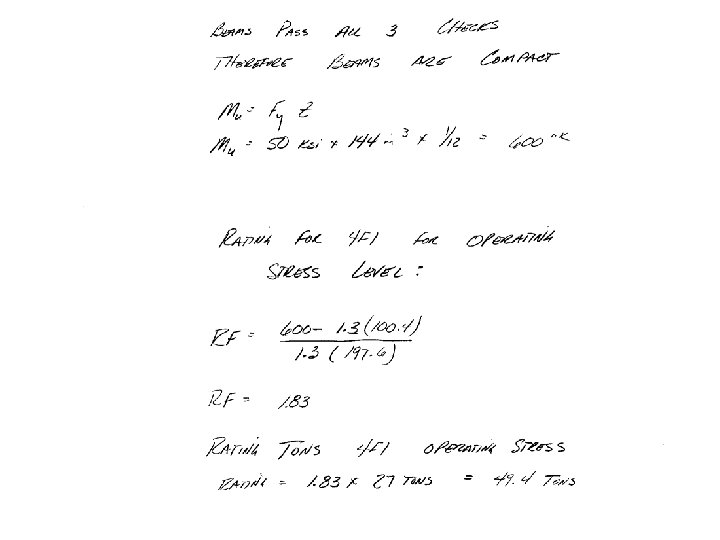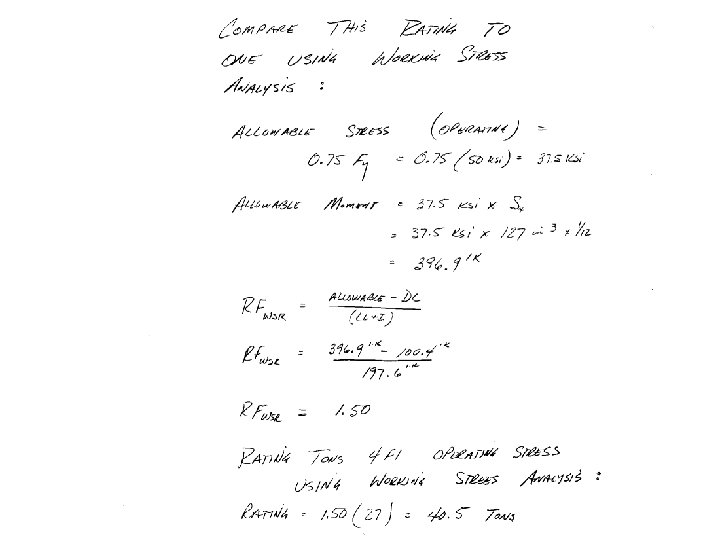Questions ? ?